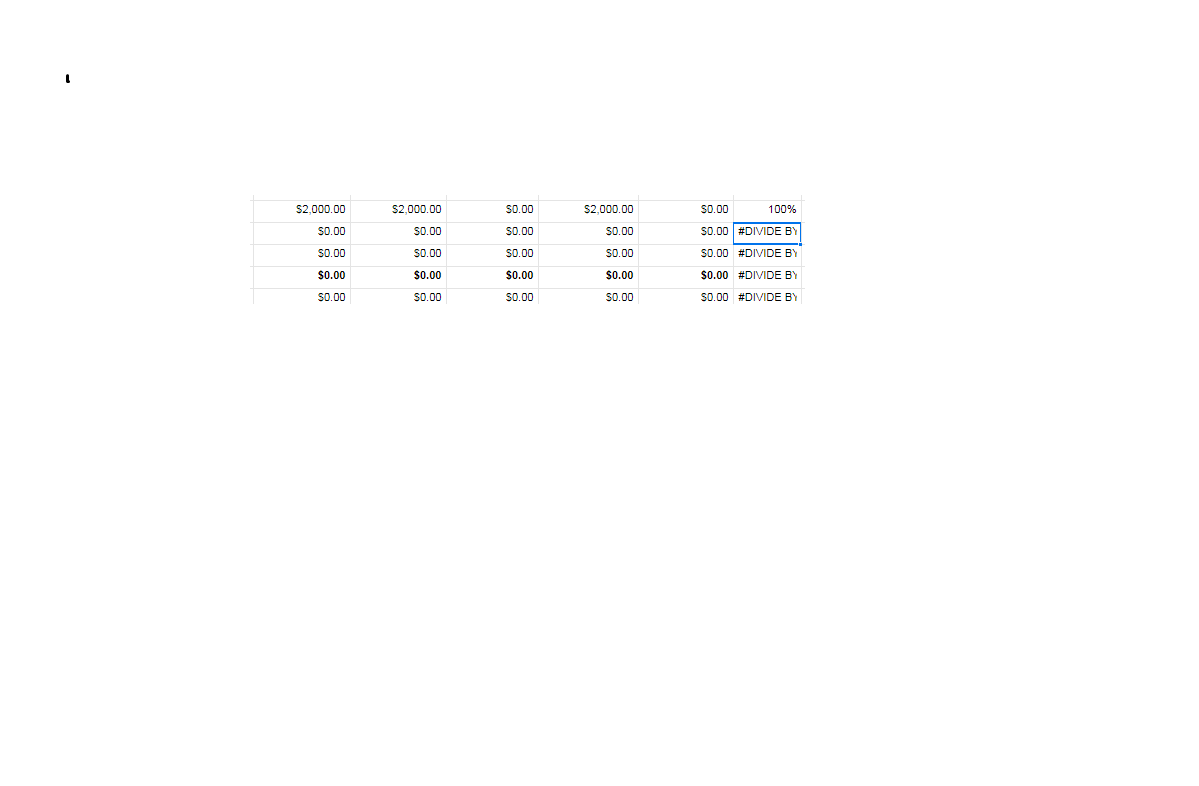# #Divide by zero error

I am having trouble with this error and need help with the formula

4 Columns (let's call them A,B, C, F)

Formula used is: =SUM(Column B + Column C)/ Column A

How do I make Column F equal Zero (0) when Column A is equal to Zero (0)

Thanks,

BM